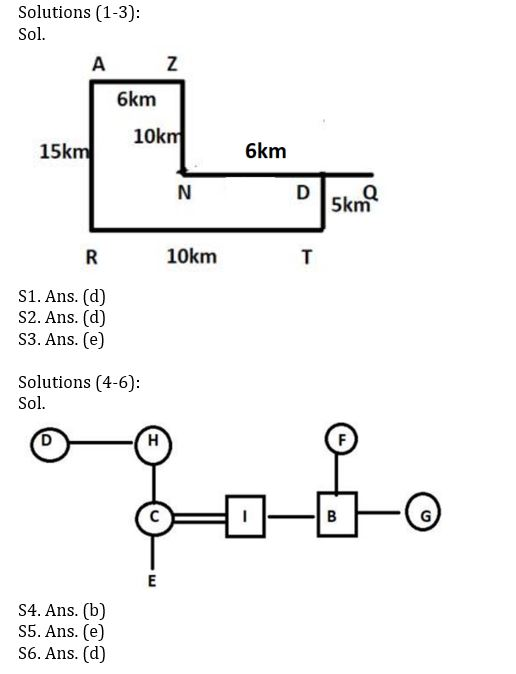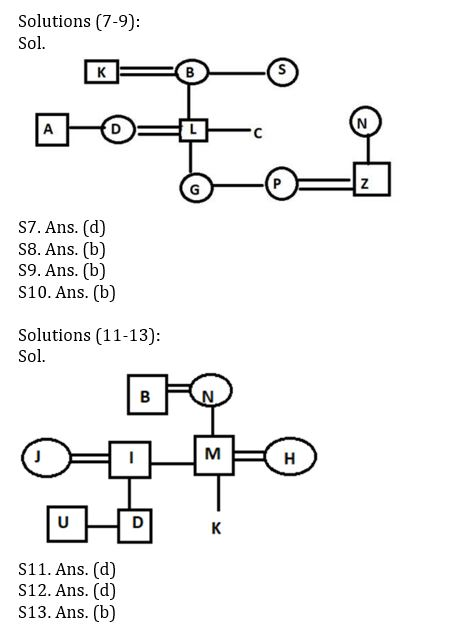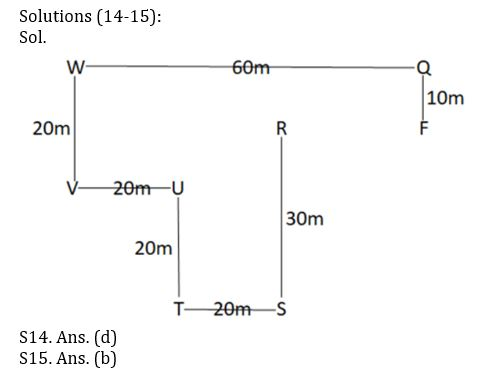Latest Banking jobs   »   Reasoning Ability Quiz For Bank Foundation...

# Reasoning Ability Quiz For Bank Foundation 2023- 15th January

Directions (1-3): Study the data carefully and answer the questions accordingly.
Mohit ran in a marathon, he started from Point Q and ran for 6km towards the west and reached Point N, then he turned towards his right and ran for 10km to reach Point Z, then he turned towards his left and ran for 6km to reach Point A, then he turned towards his left and ran for 15km to reach Point R, after that he ran for 10km towards east and reached Point T, finally took a left turn and ran for 5km to reach Point D.

Q1. What is the direction of Point D with respect to Point Z?
(a) South-west
(b North-west
(c) North
(d) South-east
(e) None of these

Q2. What is the shortest distance between Point D and Point N?
(a) 6km
(b) 18km
(c) 12km
(d) 4km
(e) None of these

Q3. What is the shortest distance between initial position of Mohit and Point R?
(a) 32km
(b) 17km
(c) 21km
(d) 37km
(e) None of these

Directions (4-6): Read the following information carefully and answer the questions given below.
A three-generation family consists of eight members B, C, D, E, F, G, H and I. C is the mother of E. I is the son-in-law of H. Only one child of F is married. G is the female member of the family. C has only one child. B is the brother-in-law of C. B is the child of F who is the mother of G. D is the maternal aunt of C. H is not a male member.

Q4. What is the relation of B with respect to E?
(a) Aunt
(b) Uncle
(c) Father
(d) Mother
(e) None of these

Q5. How many female members are there in the family?
(a) Two
(b) Three
(c) One
(d) Four
(e) Cannot be determined

Q6. If N is the husband of H, then what is the relation of N with respect to I?
(a) Aunt
(b) Mother-in-law
(c) Sister-in-law
(d) Father-in-law
(e) None of these

Directions (7-10): Read the following information carefully and answer the questions given below.
There are eleven members in a family of three generations in which there are three married couples. A is the brother of D who is the sister-in-law of C. Z is not the spouse of D. Sibling of G is the daughter-in-law of N. S is unmarried. N is the mother of Z. L is one of the members of the family. P is the female member of the family. K is the father-in-law of D. G is the granddaughter of B who is the spouse of K. G is the daughter of D. S is the sister-in-law of K.

Q7. How many female members are there in the family?
(a) Six
(b) Seven
(c) Eight
(d) Either six or seven
(e) None of these

Q8. What is the relation of P with respect to N?
(a) Son-in-law
(b) Daughter-in-law
(c) Son
(d) Daughter
(e) None of these

Q9. Who is the grandmother of G?
(a) K
(b) B
(c) S
(d) A
(e) None of these

Q10. What is the relation of L with respect to Z?
(a) Son-in-law
(b) Father-in-law
(c) Son
(d) Father
(e) None of these

Directions (11-13): Read the following information carefully and answer the questions given below.

There are nine family members i.e., D, K, M, N, J, I, U, H and B in a three-generation family in which three are married couples. J is the sister-in-law of M and D is the brother of U. U is the nephew of M, who is the father of K. H is the daughter-in-law of B. B is the spouse of N, who is the mother of I. I is married to J.

Q11. What is the relation of K with respect to B?
(a) Grandson
(b) Son
(c) Granddaughter
(d) Either grandson or granddaughter
(e) Daughter

Q12. How many female members are there in the family?
(a) Three
(b) Four
(c) Five
(d) Cannot be determined
(e) Six

Q13. What is the relation of M with respect to D?
(a) Father
(b) Uncle
(c) Brother
(d) Son
(e) None of these

Directions (14-15): Study the data carefully and answer the questions accordingly.
Point S is 30m south of point R. Point Q is 60m east of point W. Point S is 20m east of point T. Point T is 20m south of point U. Point V is 20m west of point U. Point F is 10m south of point Q. Point W is 20m north of point V.

Q14. Point R is in which direction of point F and what is the shortest distance between them?
(a) South, 20m
(b) North-East, 10m
(c) East, 30m
(d) West, 20m
(e) None of these

Q15. Point S is in which direction of point V?
(a) West
(b) South-East
(c) North-West
(d) East
(e) None of these

Solutions## FAQs

### Q1. What is the direction of Point D with respect to Point Z?

Q1. What is the direction of Point D with respect to Point Z?

#### Congratulations!Union Budget 2023-24: Free PDF